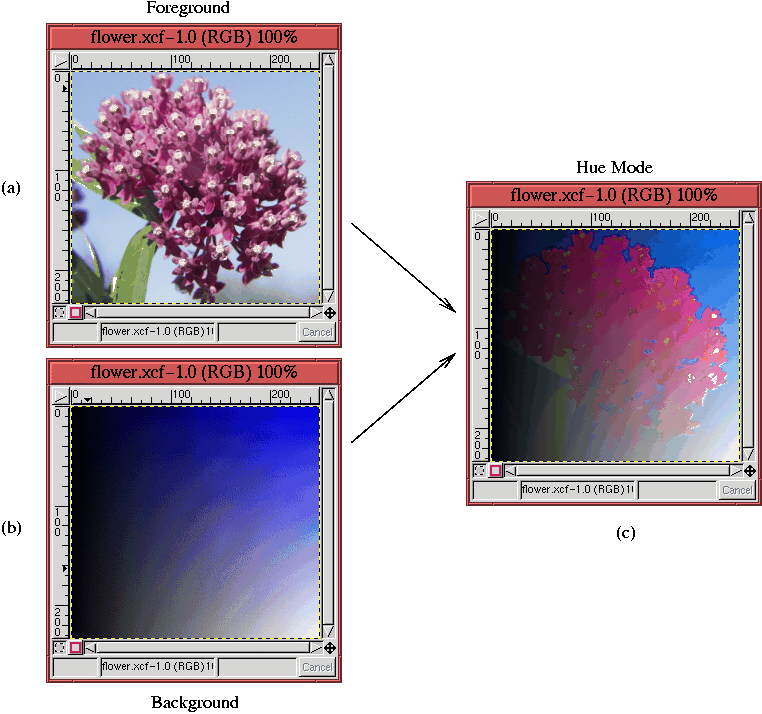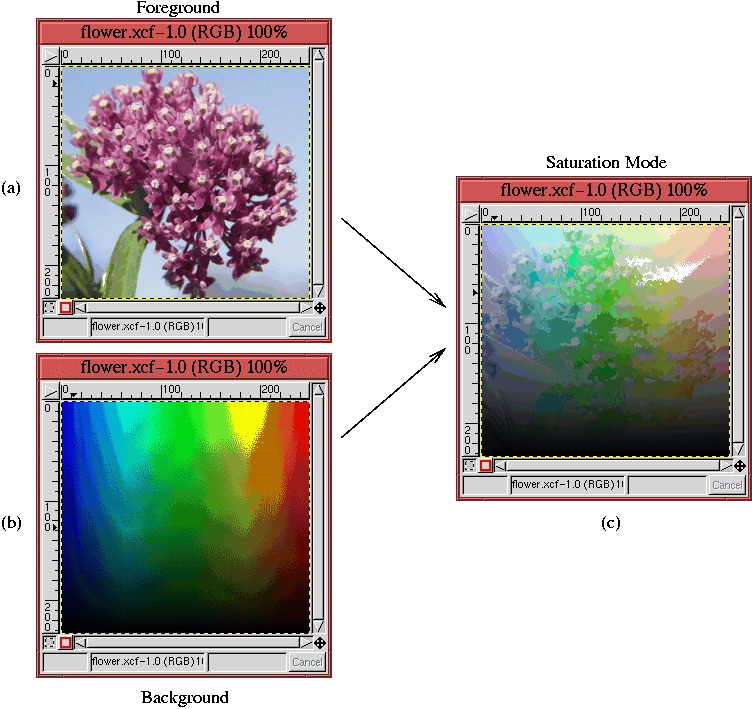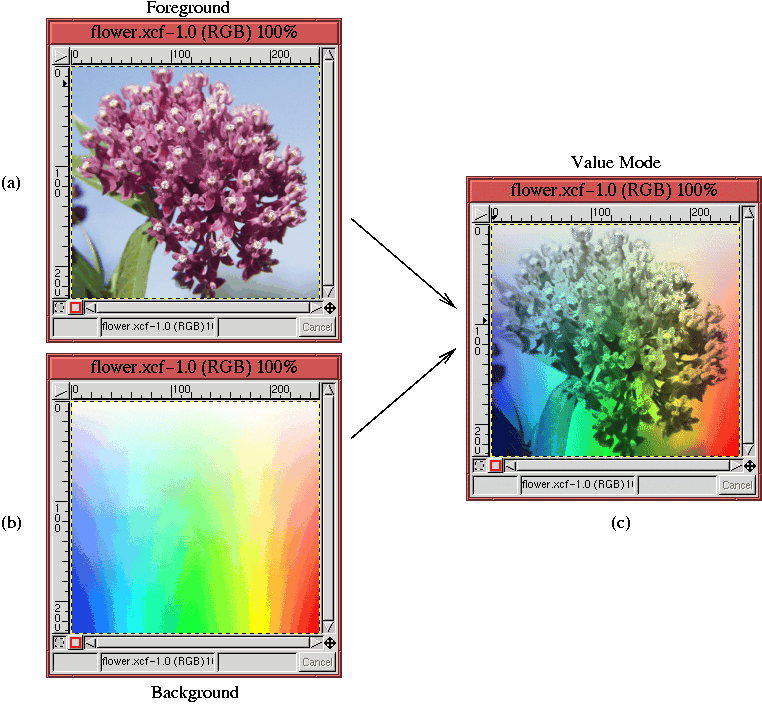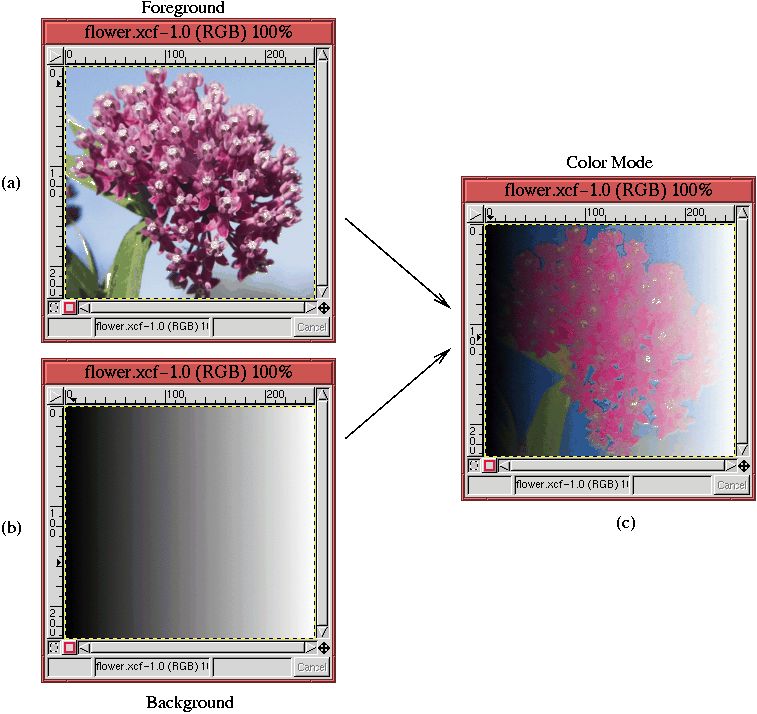On-line GuidesAll GuideseBook StoreiOS / AndroidLinux for BeginnersOffice ProductivityLinux InstallationLinux SecurityLinux UtilitiesLinux VirtualizationLinux KernelSystem/Network AdminProgrammingScripting LanguagesDevelopment ToolsWeb DevelopmentGUI Toolkits/DesktopDatabasesMail SystemsopenSolarisEclipse DocumentationTechotopia.comVirtuatopia.comHow To GuidesVirtualizationGeneral System AdminLinux SecurityLinux FilesystemsWeb ServersGraphics & DesktopPC HardwareWindowsProblem SolutionsPrivacy PolicyGrokking The Gimp
Previous Page Home Next Page

## 5.6.5 The Hue, Saturation, Value, and Color Blending Modes

The Hue, Saturation, Value, and Color blending modes all work similarly. For each mode, one HSV component is taken from the foreground pixel and the other two components from the background pixel. For example, if the Hue blending mode is chosen, the result is the hue of the foreground pixels combined with the saturation and value of the background pixels. The same process is used for the Saturation and Value blending modes. The Color blending mode, however, is slightly different. For this mode, the hue and saturation of the foreground pixels are used in conjunction with the lightness of the background pixels. Lightness, defined in Section  5.3, is less bright than value, and is simultaneously more true to the human perception of brightness.

Thus, the action of the Hue blending mode can be expressed as

R=[h(F),s(B),v(B)]

where h(F) means the hue of the foreground, s(B) represents the saturation of the background, and v(B) is the value of the background. An example of applying the Hue blending mode is illustrated in Figure  5.20.The flower image from Figure  5.15(a) is shown in Figure  5.20(a). This image is the foreground to the Hue mode, and the background is the blue layer shown in Figure  5.20(b). This blue layer varies horizontally in value and vertically in saturation. The result of applying the Hue mode is shown in Figure  5.20(c). Here, it can be clearly seen that the saturation and value variations of the blue layer are combining with the hue of the flower layer.

Similar to Hue, the Saturation blending mode produces resultant pixels that are a combination of the saturation of the foreground and the hue and value of the background. The expression for this is

R=[h(B),s(F),v(B)]

where s(F) is the saturation of the foreground, h(B) is the hue of the background, and v(B) represents the value of the background. An example of applying the Saturation blending mode is illustrated in Figure  5.21.We see again our flower image in Figure  5.21(a) playing the role of the foreground layer. However, now the background layer, shown in Figure  5.21(b), has been constructed to vary only in hue and value. In this layer, the hue changes along the horizontal direction and the value along the vertical. The result of applying the Saturation mode is shown in Figure  5.21(c).

The Value blending mode produces resultant pixels that are a combination of the value of the foreground and the hue and saturation of the background. The expression for this is

R=[h(B),s(B),v(F)]

Here, v(F) represents the foreground value, h(B) the background hue, and s(B) the background saturation. An example of this blending mode is shown in Figure  5.22.The background layer, shown in Figure  5.22(b), varies in hue in the horizontal direction and saturation in the vertical direction. The result of this mode is shown in Figure  5.22(c).

The final example in this section illustrates the Color blending mode. This mode combines the foreground hue and saturation with the background lightness. Lightness was defined earlier in Section  5.3; lightness is always a bit less bright than value. The expression for this blending mode is

R=[h(F),s(F),l(B)]

where h(F) and s(F) are the hue and saturation of the foreground, and l(B) is the lightness of the background. An example of this blending mode is shown in Figure  5.23.Here, the background, shown in Figure  5.23(b), varies only in value. The result is shown in Figure  5.23(c).